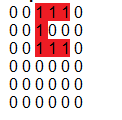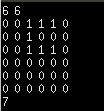Q:

# Find the perfect land of C shape

Debasis is searching for a perfect land to build a new house. There is an area of size N*M and each point of N*M has a value. But he wants exactly a land of size 3*3 but the land should be perfect.

perfect land is a land whose 'C' shape value is maximum.

You being his friend, help him to find the perfect land.

Input

First line of the input is two space separated integer N and M

Second line of the input contains the matrix of size N*M (N rows M columns).

Output

A single integer containing the sum of the perfect land.

Example:

```    Input:
6 6
0 0 1 1 1 0
0 0 1 0 0 0
0 0 1 1 1 0
0 0 0 0 0 0
0 0 0 0 0 0
0 0 0 0 0 0

Output:
7```Here is the perfect land whose sum value is maximum.

Note: Before going to solution please try it by yourself.

C++ implementation:

``````#include <bits/stdc++.h>
#define FASTIO ios_base::sync_with_stdio(false); cin.tie(NULL)

using namespace std;
int main()
{
FASTIO; //Taking Fast Input Output
int n,m,x;
cin>>n>>m;      //taking input n and m

int arr[n][m];
for(int i=0;i<n;i++)
{
for(int j=0;j<m;j++)
{
//taking input for the matrix
cin>>arr[i][j];
}
}

//it will store the maximum value of the perfect land
int max1=0;
for(int i=0;i<n-2;i++)
{
for(int j=0;j<m-2;j++)
{
//it will calclute the total sum of each C shape land
int sum1=0;
//here we are calculating the sum of each C shape
//land of size 3*3
//this is for first line of C shape
sum1=sum1+arr[i][j]+arr[i][j+1]+arr[i][j+2];
//second line of C shape
sum1+=arr[i+1][j];
//3rd line of C shape
sum1+=arr[i+2][j]+arr[i+2][j+1]+arr[i+2][j+2];

//checking if the sum is grater than
//the previous C shape land
if(sum1>=max1)
max1=sum1;
}
}
cout<<max1<<"\n";

return 0;
}``````

Output